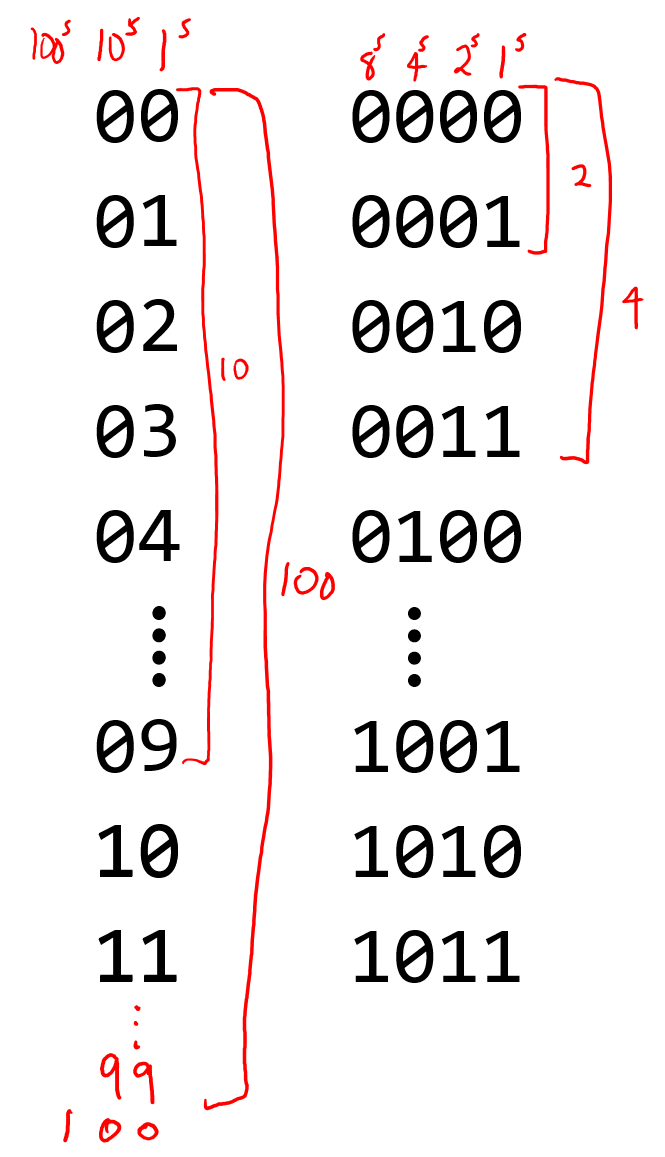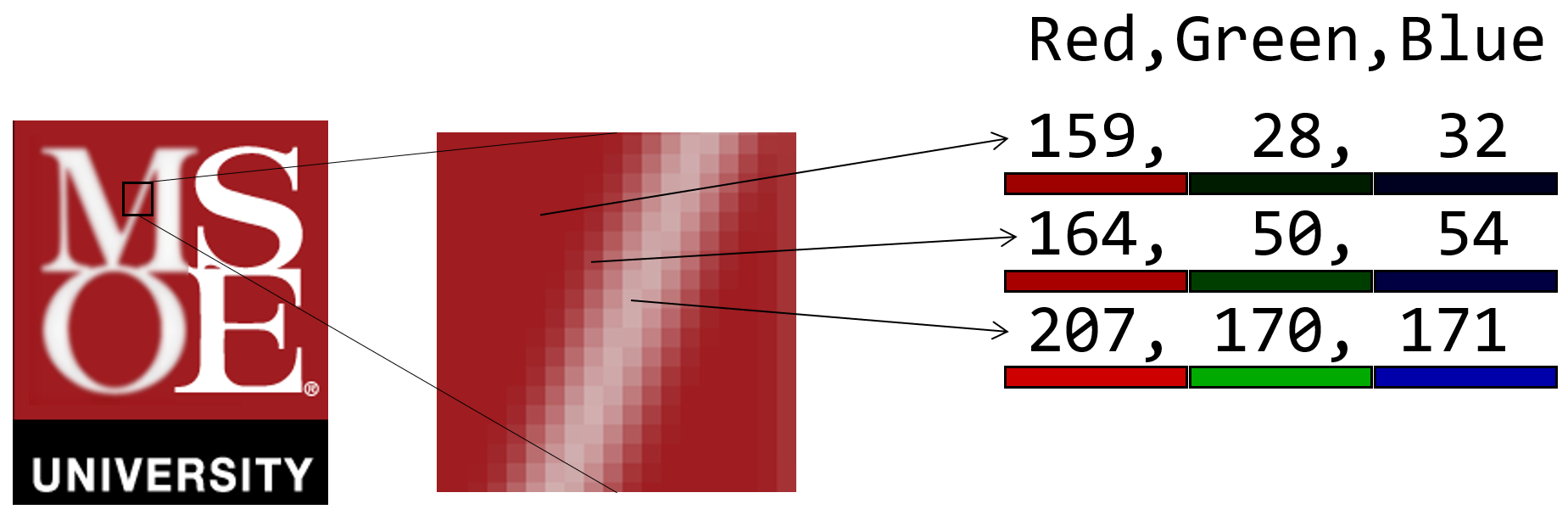taylorialcom/ Fundamentals

# How Computers Think

## Storing Information### Digital Systems - Binary

• Digital systems consist of a collection of switches
• Each switch represents one of two states: 0-1, on-off, true-false, ...
• The hardware switches may be one of the following:

### Unsigned Integers

• In the base-ten number system we have ten different symbols to represent things.
• If we need to represent more than ten things, we add a second digit.
• The right most column represents the $$1^s$$ place
• The column just to the left of it represents the $$10^s$$ place since for each symbol in the second column, we have $$10$$ possibilities
• The left side of the figure below illustrates this
• The right side of the figure illustrates binary (base-two) numbers• In the base-two number system we have two different symbols to represent things.
• If we need to represent more than two things, we add a second digit.
• The right most column represents the $$1^s$$ place
• The column just to the left of it represents the $$2^s$$ place since for each symbol in the second column, we have $$2$$ possibilities
• The column third from the right represents the $$4^s$$ place since for each symbol in the third column, we have $$4$$ possibilities

### Signed Integers

• If we wish to represent negative values, we need to come up with a different technique for assigning meaning to the sequences of ones and zeros.
• One possibility would be to use the most significant bit (the column furthest to the left) to represent the sign of the number.
• In the table below, numbers that begin with a $$0$$ are just like a 3-bit unsigned integer.
• Numbers that begin with a $$1$$ are considered negative.
• Here you can think of the sequence of numbers wrapping around like an odometer. One larger than $$1111$$ is $$0000$$. Since $$0000$$ is $$0$$, one less than $$0000$$ is $$-1$$.
BinaryDecimal
$$1000$$$$-8$$
$$1001$$$$-7$$
$$1010$$$$-6$$
$$1011$$$$-5$$
$$1100$$$$-4$$
$$1101$$$$-3$$
$$1110$$$$-2$$
$$1111$$$$-1$$
$$0000$$$$0$$
$$0001$$$$1$$
$$0010$$$$2$$
$$0011$$$$3$$
$$0100$$$$4$$
$$0101$$$$5$$
$$0110$$$$6$$
$$0111$$$$7$$

### Letters/Symbols

• We represent letters and symbols using a standard mapping to a binary sequence.
• Two popular mappings are ASCII and UNICODE.

### Colors/Images

• Colors can be stored as a combination of three integer values between 0 and 255.
• Each integer represents the intensity of colored light.
• With the appropriate mixture of Red, Green, and Blue light we can reproduce most colors.
• This is a convenient way to represent colors since Flat Panel Displays produce colors as a combination of Red, Green, and Blue components.
• More details on RGB Colors## Acting on Information

### Truth Tables

Suppose we wish to add two numbers, $$a$$ and $$b$$, together. For simplicity, we'll limit the range of values for these number between $$0$$ and $$3$$. We can list every possible combination of input values and the corresponding result, $$c$$ (left two columns of the table below). The right two columns contain the binary representation for adding two 2-bit numbers together.

$$a + b$$$$c$$<- Decimal ... Binary ->$$a_2 a_1 b_2 b_1$$$$c_4 c_2 c_1$$
$$0 + 0$$$$0$$$$0000$$$$000$$
$$0 + 1$$$$1$$$$0001$$$$001$$
$$0 + 2$$$$2$$$$0010$$$$010$$
$$0 + 3$$$$3$$$$0011$$$$011$$
$$1 + 0$$$$1$$$$0100$$$$001$$
$$1 + 1$$$$2$$$$0101$$$$010$$
$$1 + 2$$$$3$$$$0110$$$$011$$
$$1 + 3$$$$4$$$$0111$$$$100$$
$$2 + 0$$$$2$$$$1000$$$$010$$
$$2 + 1$$$$3$$$$1001$$$$011$$
$$2 + 2$$$$4$$$$1010$$$$100$$
$$2 + 3$$$$5$$$$1011$$$$101$$
$$3 + 0$$$$3$$$$1100$$$$011$$
$$3 + 1$$$$4$$$$1101$$$$100$$
$$3 + 2$$$$5$$$$1110$$$$101$$
$$3 + 3$$$$6$$$$1111$$$$110$$

•                                                      *

• A1-----*--.__..---. *
•     |   ___))   )------------------------------- C1  *

• B1---*-+--' ''---' *
•   | |                                                *

•   | '--.__.---.                                      *

•   '----.__|    )------.                              *

•           '---'       |                              *

•                       |                              *

• A2-----*--...---. +--+--...---. *
•     |   ___))   )--*  |   ___))   )------------- C2  *

• B2---*-+--' ''---' | *--' ''---' *
•   | |              |  |                              *

•   | '--.__.---.    +--+--.__.---.                    *

•   '----.__|    )--.   '--.__|    )--.__.---.         *

•           '---'   |         '---'    ___)   )--- C4  *

•                   '-----------------'  '---'         *

•                                                      *


Computer engineers have techniques for building integrated circuits that replicate this input-output behavior. The figure above shows such a circuit. It is composed of a collection of logic gates that each take two inputs and produce one output.

The circuit above uses three types of logic gates that each have their own input-output relationship.

#### AND Gate

• X __.---. *
• Y __| )-- Z *
• '---'      *


XYZ
000
010
100
111

#### OR Gate

• X __.---. *
• Y ___) )-- Z *
• '---'      *


XYZ
000
011
101
111

#### XOR Gate

• X __..---. *
• Y ___)) )-- Z *
• ''---'      *


XYZ
000
011
101
110

We could create a similar table for multiplying two 2-bit numbers together. In this case the result could be as large as $$9$$, so we need four bits to represent the output:

$$a \times b$$$$c$$<- Decimal ... Binary ->$$a_2 a_1 b_2 b_1$$$$c_8 c_4 c_2 c_1$$
$$0 \times 0$$$$0$$$$0000$$$$0000$$
$$0 \times 1$$$$0$$$$0001$$$$0000$$
$$0 \times 2$$$$0$$$$0010$$$$0000$$
$$0 \times 3$$$$0$$$$0011$$$$0000$$
$$1 \times 0$$$$0$$$$0100$$$$0000$$
$$1 \times 1$$$$1$$$$0101$$$$0001$$
$$1 \times 2$$$$2$$$$0110$$$$0010$$
$$1 \times 3$$$$3$$$$0111$$$$0011$$
$$2 \times 0$$$$0$$$$1000$$$$0000$$
$$2 \times 1$$$$2$$$$1001$$$$0010$$
$$2 \times 2$$$$4$$$$1010$$$$0100$$
$$2 \times 3$$$$6$$$$1011$$$$0110$$
$$3 \times 0$$$$0$$$$1100$$$$0000$$
$$3 \times 1$$$$3$$$$1101$$$$0011$$
$$3 \times 2$$$$6$$$$1110$$$$0110$$
$$3 \times 3$$$$9$$$$1111$$$$1001$$

Your computer's CPU (central processing unit) contains a number of these these pre-built circuits for doing integer math and math for numbers with a decimal point (floating point math), along with other circuits for manipulating binary inputs.

Ray tracing, video rendering, and artificial intelligence problems require massive amounts of computation. GPUs (graphical processing units) contain many copies of a limited set of circuit types than CPUs. Software that is written to exploit massively parrallel operations can run exteremely fast compared to traditional CPU processing.

The circuits in CPUs and GPUs serve as the foundation for the software we create.